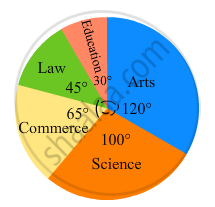Share

# The Following Pie-chart Shows the Number of Students Admitted in Different Faculties of a College. If 1000 Students Are Admitted in Science Answer the Following: - Mathematics

Course
ConceptIntroduction of Circle Graph Or Pie Chart

#### Question

The following pie-chart shows the number of students admitted in different faculties of a college. If 1000 students are admitted in Science answer the following:(i) What is the total number of students?
(ii) What is the ratio of students in science and arts?

#### Solution

(i) $\text{ Students in science }= \frac{\text{ Central angle of the corresponding sector }\times\text{ Total students }}{360^\circ}$
$1000 = \frac{100^\circ \times\text{ Total students }}{360^\circ}$
$\therefore\text{ Total students }= 3600$

(ii)$\text{ Students in arts }= \frac{\text{ Central angle for arts }\times\text{ Total students }}{360^\circ}$

$= \frac{120^\circ \times 3600}{360^\circ} = 1200$

$\therefore\text{ Ratio of students in science and arts }= 1000: 1200 = 5: 6$

Is there an error in this question or solution?

#### APPEARS IN

RD Sharma Solution for Mathematics for Class 8 by R D Sharma (2019-2020 Session) (2017 to Current)
Chapter 25: Data Handling-III (Pictorial Representation of Data as Pie Charts or Circle Graphs)
Ex. 25.2 | Q: 3 | Page no. 22

#### Video TutorialsVIEW ALL 

Solution The Following Pie-chart Shows the Number of Students Admitted in Different Faculties of a College. If 1000 Students Are Admitted in Science Answer the Following: Concept: Introduction of Circle Graph Or Pie Chart.
S